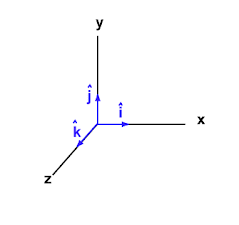Thus far, we have examined various aspects of one-dimensional motion; i.e. motion in a straight line aligned with one of the axes on our coordinate system. But it's quite obvious that much of what moves in the world cannot be modeled this way (e.g. a baseball flying through the air). We now lay the groundwork that will enable us to model two-dimensional motion (which is common, despite living within a 3D world).

As stated before, any quantity that can be measured and that includes (or could include) a direction, like velocity, is called a vector quantity.

Such quantities are often depicted on Cartesian coordinate systems as arrows (see below). The length of the arrow signifies the magnitude or size of the quantity and the direction its pointing determines, well, the direction of the quantity.

These arrows are themselves called vectors. They represent vector quantities.

Below, you see the depiction of four vectors. Each of these vectors (let's refrain from saying arrows) represents some measurable quantity.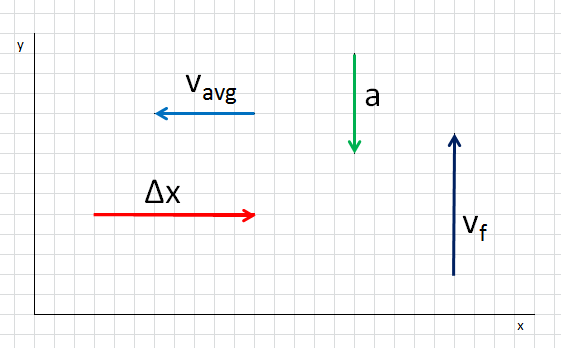For example. the light blue vector could represent some object's average velocity over some period of time. Perhaps a car is driving westward at 4 m/s and it speeds up to 6 m/s, in a time of 2 seconds, after which it maintains its speed of 6 m/s for an additional second. During those first two second, the car has an average velocity of -5 i (m/s).

The vector is a way of depicting some aspect of the motion; in this case, it depicts the average velocity over the first two seconds of the car's documented motion. Why would you want to depict this part of its motion with a vector? Maybe you wouldn't! But there are times when vectors are very useful, and you'll see examples later.

Let's make something very clear. A vector is NOT a graph of our equation of motion. I don't know if that thought even crossed your mind, but they are different things.

Notice, a graph is a collection of data points, often connected by a line or curve. A vector is a single arrow with a certain length and direction. Both graphs and vectors are useful tools. You should already understand how a graph can be useful for modeling motion. As stated before, we'll soon encounter examples that demonstrate the usefulness of vectors.

Alright, before moving on, let's comment on the other three vectors depicted above (and again at left).

The red vector represents a displacement in the i direction. We could say it is the vector associated with ∆x = 8 i (m). It's not really appropriate to call this equation a model. Rather, it is a model evaluated at a certain time.

Maybe the equation of motion is ∆x = (1 i)t2 + (2 i)t and you're wanting to find the displacement at t = 2 seconds. Substituting t = 2 into the function would result in ∆x = 8 i (m).

Moving on, the green vector could represent a = -5 j (m/s2).

And the dark blue vector could represent vf = 7 j (m/s).

Or the green vector could represent a = -50 j (m/s2) and the dark blue vector could represent vf = 70 j (m/s). To accurately state the magnitude of a vector, based on a drawing, you must know the scale of the drawing. This is no different than interpreting distances on a map.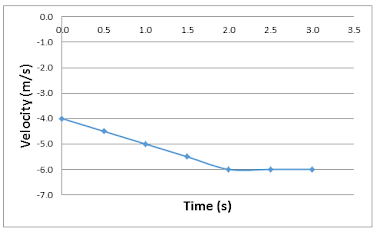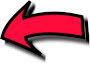Here is the equation of motion.

vf = (-1 i)t + (-4 i), 0t2

vf = -6 i2t3

And here is its graph.

(You would have to look to the underlying data to be able to plot this.)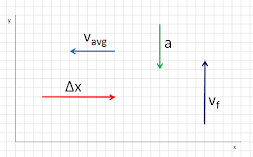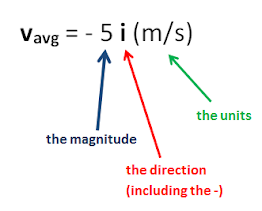Equation form of vector. (You already know this.)When drawing multiple vectors on the same grid, use a consistent scale.

If this vector represents 10 i (m)...

then this vector should represent 20 i (m). It should be twice as long.

And this vector should represent -6 i (m).

This means that i by itself represents a vector of length 1, otherwise called a unit vector.

And j is a unit vector pointing (typically) upward.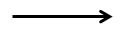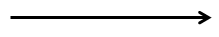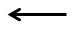I've spent some time giving you a conceptual overview of vectors. As we move forward and use them, you'll become more comfortable with them. (Again, they are a tool. We only bother dealing with them because they'll make modeling the world easier.)

It's useful to be able to add vectors together. (Yes, there's a whole branch of mathematics built around vectors. Just like with numbers, we can add them, subtract them, and even multiply them.) Let's see a few examples of vector addition. Note that they are 2-dimensional pictures.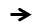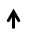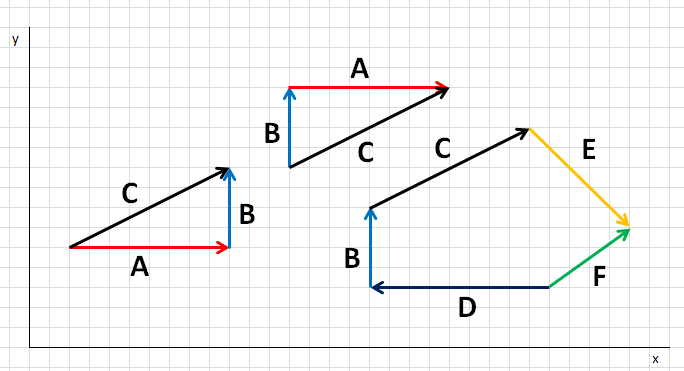We see that:

A + B = C

B + A = C

D + B + C + E = F

Geometrically, vector addition involves arranging the vectors head to tail. To add B to A, place the tail of B at the head of A. The "answer" or sum is a new vector, drawn from the tail of the first vector in the chain, A, to the head of the final vector in the chain, B. So, for the leftmost example above, C is the "answer" you get when you sum A + B.

Just as with adding numbers, the order in which you add vectors does not affect the result. Both A + B and B + A are equal to C. Verify this above. Remember, a vector is characterized by two things: its length or magnitude and its direction. Each instance of C above has the same magnitude and direction.

3 + 5 = 8    analogously    A + B = C

5 + 3 = 8    analogously    B + A = C

When you look at the sum D + B + C + E = F above, is it obvious from the picture alone that F is the sum? It should be. F stands out because it is the only vector that isn't head to tail. Rather, it points from the "initial tail" to the "final head".

Let's associate equations with these geometric representations of vector addition.

I'm going to omit units for now, so they don't clutter things up. As stated before, these are not the equations of motion, but they are equations.

A = 8 i

B = 4 j

C = 8 i + 4 j

D = -9 i

B = 4 j

C = 8 i + 4 j

E = 5 i - 5 j

F = 4 i + 3 j

Let's prove that adding D + B + C + E does result in FTo sum these vectors, simply add up all of the i terms, which becomes the i term of the result. Then add up all the j terms, which becomes the j term of the result.

F = (-9 + 8 + 5)i + (4 + 4 - 5)j = 4 i + 3 j

Don't breeze past this part. Really think about this summation and why adding up all the i terms and j terms gives us the terms of the resultant vector. Feel it in your bones, if you can, before moving on.

If you wanted to know the length of C, could you find it? Try to work it out before reading on.You find the length of C using the Pythagorean Theorem.

The absolute value bars around the C indicate that I'm evaluating its magnitude, ignoring its direction. To find its direction, you'd need trigonometry. We'll get to that later.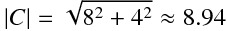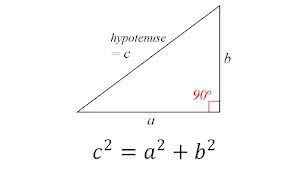Before moving on, I want to give you one example of how vector addition can help us to model motion.

Imagine that each of the vectors D, B, C and E represent a displacement by a child. The child walks 9 m west, then 4 m north, then 8 m east and 4 m north, then 5 m east and 5 m south. The sum of those displacements (F) gives us the child's overall displacement.

If the various displacements took 25 seconds, what was the child's average velocity?

Adding the various displacements results in F = 4 i + 3 j.

As average velocity = displacement / time, we have:

vavg = (4 i + 3 j) / 25 = .16 i + .12 j (m/s)

If you wanted the magnitude of the average velocity, the Pythagorean Theorem would return:

The child had an average velocity of .2 m/s in some particular direction (the direction of F, which we'd have to find using trigonometry).

Okay, let's look at another complete example, and then you'll be ready to practice manipulating vectors. Soon enough, you'll be using them to model motion and (even later) various other aspects of the world.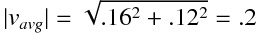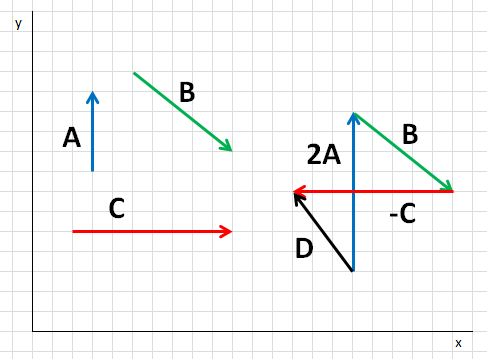A = 4 j

B = 5 i - 4 j

C = 8 i

2A + B - C = D

Let's solve for D.

2(4 j) + (5 i - 4 j) - (8 i) = (5 - 8)i + (8 - 4)j = -3 i + 4 j

Therefore, D = -3 i + 4 j, which checks out with the geometric representation above.

Vector subtraction is straightforward using equations. Geometrically, subtraction involves rotating by 180° the vector to be subtracted, then linking it to the other vectors in the chain the same as we do for addition. We do this above with C. Post-flipping, we label it -C. Realize that 5 - 3 is the same as 5 + (-3); i.e. subtraction is a form of addition. Above, we have 2A + B + (-C) = D.

Practice  problems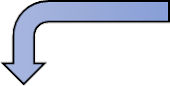In order to find the direction of B, we must think of it as the hypotenuse of a right triangle. I've redrawn B away from the vector sum, to avoid clutter, and I've shaded part of the graph paper in yellow to help you visualize a right triangle, for which B is the hypotenuse. (Yes, you could have created the triangle on the other side of B. It doesn't matter.)

I've placed a compass rose at the tail of the vector, as you should always imagine doing. (You can just imagine doing it. You don't have to literally draw it in.) B is pointing more-or-less southeast, according to the compass rose. We want to know the specific angle the vector B makes with an axis (usually the nearest axis; here, East).

Going forward, we'll use trigonometry.

First, we'll assume that rightward is eastward. That is, unit vector i points eastward. And upward is northward; unit vector j points northward.

Let's find the directions of vectors B and D, relative to the cardinal directions: North-South-East-West.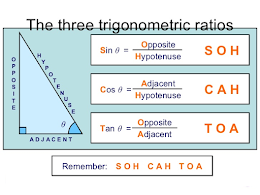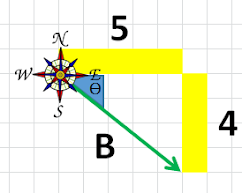I've redrawn B here, and I've labeled the lengths of the two sides of the imagined triangle. You would know these two lengths from the equation for B, shown below. On graph paper, you could just measure them.

B = 5 i - 4 j

We want to find the angle ϴ (theta), shaded in blue in the drawing. We know that:

Therefore,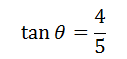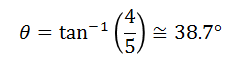So here's our answer. The vector B is pointing 38.7° south of east. Some people write this as [E38.7°S], which can be read as "East 38.7° South".

(This is equivalent to saying 51.3° east of south. Note: these two angles are complements, adding to 90°.)

And now for vector D.

The angle tucked up in the corner next to the compass rose, inside the imagined triangle, is the one we want. We call it ϴ (theta).

Thus, the direction of D is 53.1° north of west. Or [W53.1°N].

(Realize this is equivalent to saying 36.9° west of north,)

(You could also state the direction as 126.9° (90° + 36.9°) from east,

rotating counterclockwise; i.e. 126.9° CCW from east.)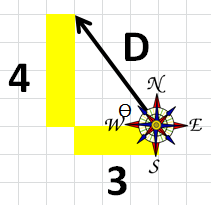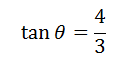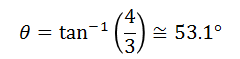Make sure your calculator is in "degree mode" when you are working with degrees!problems

*Hieggelke, C. J., Maloney, D. P., & Kanim, S. E. (2012). Newtonian tasks inspired by physics education research: NTIPERs. Boston: Addison-Wesley.

-nTIPERs* worksheets

Forces

Kinematics